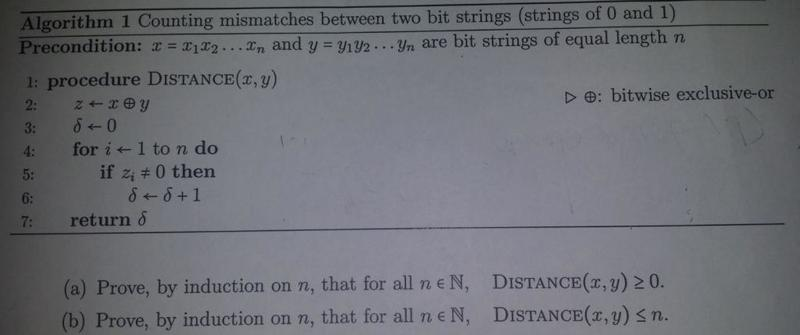# Proof, by induction on n

Jtechguy21

## Homework Statementa)Prove, by induction on n, that for all n ∈ N(natural numbers), Distance(x,y)<or equal to 0

b)Prove, by induction on n, that for all n ∈ N(natural numbers), Distance(x,y)<or equal to n

## Homework Equations

proof by induction:

Base case P(1)

then assume p(k) is true
then prove p(k+1) is also true by induction.

## The Attempt at a Solution

Alright so I have been practicing induction problems in my discrete mathematics class,
however I am not quite sure where to start.
I just need some guidance to starting the problem. (the algorithm throws me off, im new to coding)
I do understand the concept of induction.

Specifically...

In part a, What exactly is the statement that I am proving by induction?
p(n)= n >(or equal to) 0 is this correct?

gopher_p

## Homework Statementa)Prove, by induction on n, that for all n ∈ N(natural numbers), Distance(x,y)<or equal to 0

b)Prove, by induction on n, that for all n ∈ N(natural numbers), Distance(x,y)<or equal to n

## Homework Equations

proof by induction:

Base case P(1)

then assume p(k) is true
then prove p(k+1) is also true by induction.

## The Attempt at a Solution

Alright so I have been practicing induction problems in my discrete mathematics class,
however I am not quite sure where to start.
I just need some guidance to starting the problem. (the algorithm throws me off, im new to coding)
I do understand the concept of induction.

Specifically...

In part a, What exactly is the statement that I am proving by induction?
p(n)= n >(or equal to) 0 is this correct?

In part (a), you're meant to show that this function, ##\mathrm{Distance}(x,y)##, assigns a non-negative value to a pair of binary strings. In order to do that, you need to first understand the function. And in order to do that, you gotta figure out what the algorithm is doing.

The first tricky bit is understanding the meaning of the ##\oplus## operator. Your book tells you that this is meant to represent "exclusive or" but that's not really all that helpful. Basically, given ##a,b\in\{0,1\}##, ##a\oplus b=0## if ##a=b## and ##a\oplus b=1## if ##a\neq b##. Or to put it another way, ##a\oplus b=|a-b|##. Or to put it yet another way - a way that gets at the heart of the "exclusive or" business - ##a\oplus b=1## if and only if either ##a=1## or ##b=1##, but not both.

Now given that, if I tell you that ##\mathrm{Distance}(x,y)=\sum\limits_{i=1}^n|x_i-y_i|##, can you figure out how the algorithm works? Note that this formula make the problem trivial, and completely doable without induction. So I give it only so that you may understand the algorithm.

Also the ##p(n)## statement for part (a) would look something like, "If ##x## and ##y## are binary strings of length ##n##, then ##\mathrm{Distance}(x,y)\geq 0##."

Staff Emeritus